# Velocity vs time graph Calculating the area under

• Slides: 33Velocity vs time graph Calculating the area under the graph Displacement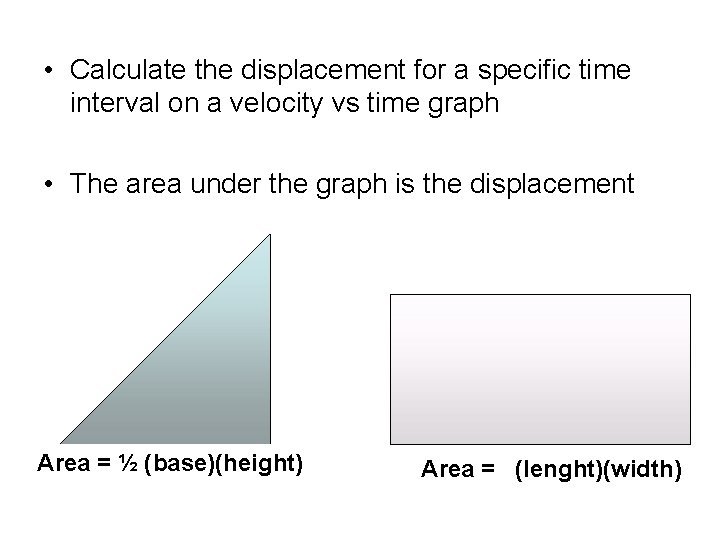• Calculate the displacement for a specific time interval on a velocity vs time graph • The area under the graph is the displacement Area = ½ (base)(height) Area = (lenght)(width)• To follow along print slides 5, 13, 20, 27Graph #1Graph 1 Determine the area under the graph for each segment +15 m/s velocity +10 m/s +5 m/s 0 m/s -5 m/s -10 m/s 0 s 1 s 2 s time 3 s 4 s 5 s 6Graph 1 Determine the area under the graph for each segment +15 m/s velocity +10 m/s +5 m/s 0 m/s -5 m/s -10 m/s 0 s 1 s 2 s time 3 s 4 s 5 s 6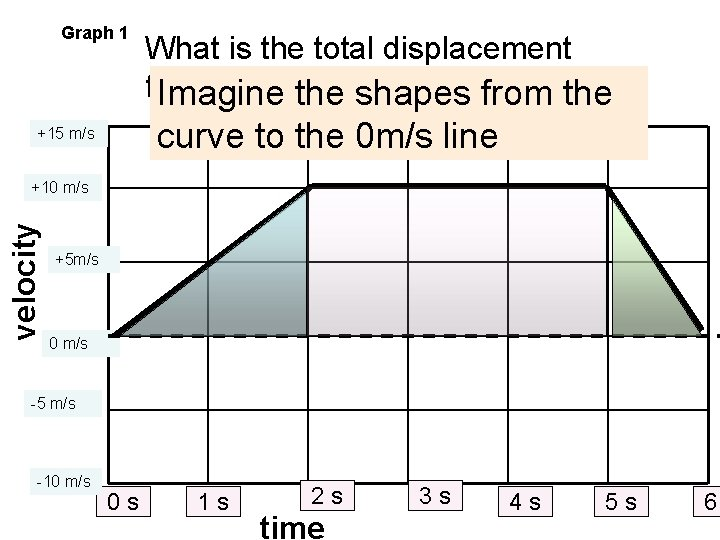Graph 1 What is the total displacement from 0 to 6 the s? shapes from the Imagine curve to the 0 m/s line +15 m/s velocity +10 m/s +5 m/s 0 m/s -5 m/s -10 m/s 0 s 1 s 2 s time 3 s 4 s 5 s 6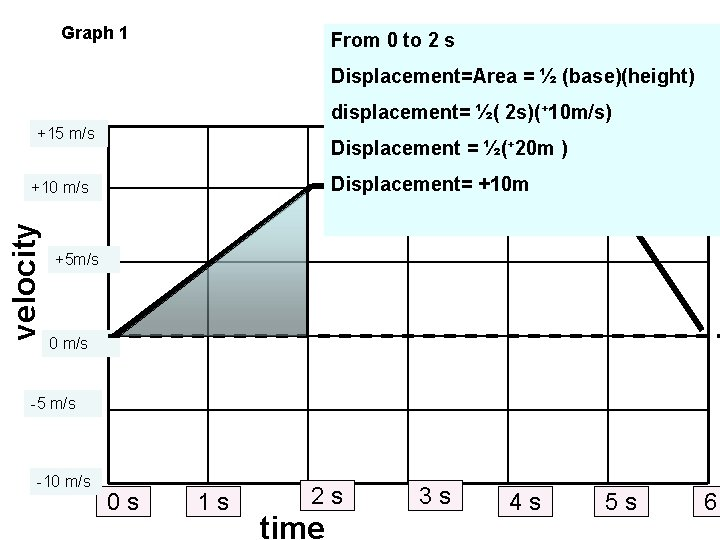Graph 1 From 0 to 2 s Displacement=Area = ½ (base)(height) displacement= ½( 2 s)(+10 m/s) +15 m/s Displacement = ½(+20 m ) Displacement= +10 m velocity +10 m/s +5 m/s 0 m/s -5 m/s -10 m/s 0 s 1 s 2 s time 3 s 4 s 5 s 6Graph 1 From 2 to 5 s Displacement=Area = (length)( width) displacement= ( 3 s)(+10 m/s) Displacement = (+30 m ) +15 m/s Displacement= +30 m velocity +10 m/s +5 m/s 0 m/s -5 m/s -10 m/s 0 s 1 s 2 s time 3 s 4 s 5 s 6Graph 1 From 5 to 6 s Displacement=Area = ½ (base)(height) displacement= ½( 1 s)(+10 m/s) +15 m/s Displacement = ½(+10 m ) Displacement= + 5 m velocity +10 m/s +5 m/s 0 m/s -5 m/s -10 m/s 0 s 1 s 2 s time 3 s 4 s 5 s 6Graph 1 What is the total displacment from 0 to 6 s? +30 m +15 m/s velocity +10 m/s + 5 m +10 m +5 m/s 0 m/s Total displacement= +10 m + +30 m+ +5 m= -5 m/s 45 m -10 m/s 0 s 1 s 2 s time 3 s 4 s 5 s 6Graph #2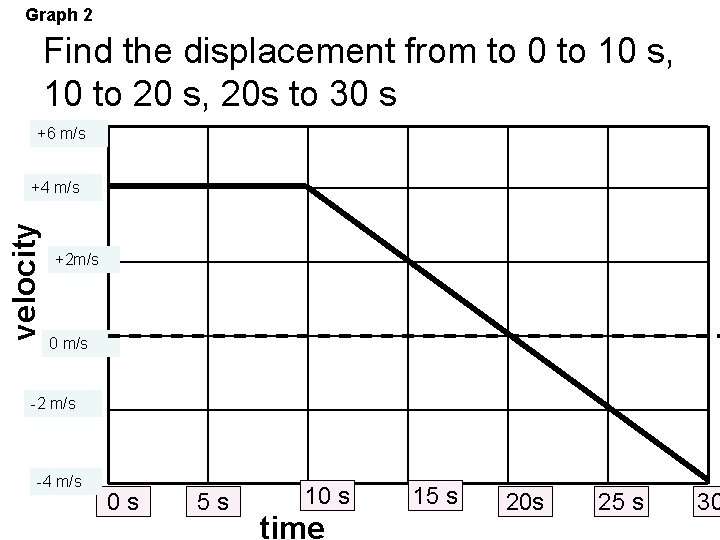Graph 2 Find the displacement from to 0 to 10 s, 10 to 20 s, 20 s to 30 s +6 m/s velocity +4 m/s +2 m/s 0 m/s -2 m/s -4 m/s 0 s 5 s 10 s time 15 s 20 s 25 s 30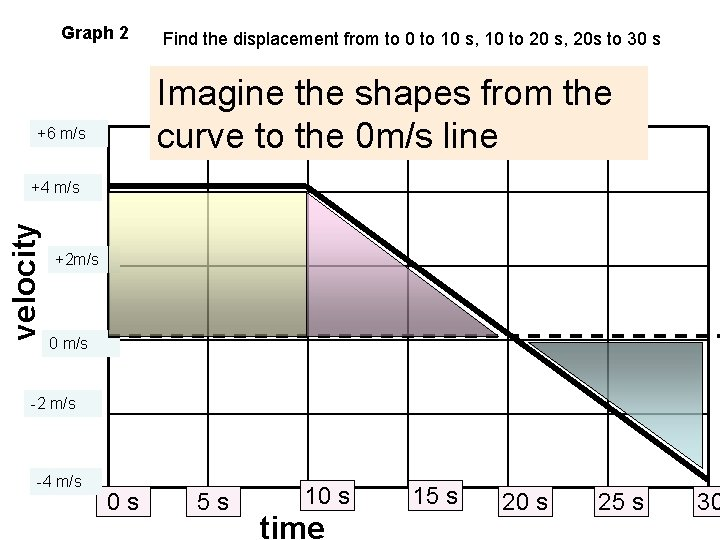Graph 2 Find the displacement from to 0 to 10 s, 10 to 20 s, 20 s to 30 s Imagine the shapes from the curve to the 0 m/s line +6 m/s velocity +4 m/s +2 m/s 0 m/s -2 m/s -4 m/s 0 s 5 s 10 s time 15 s 20 s 25 s 30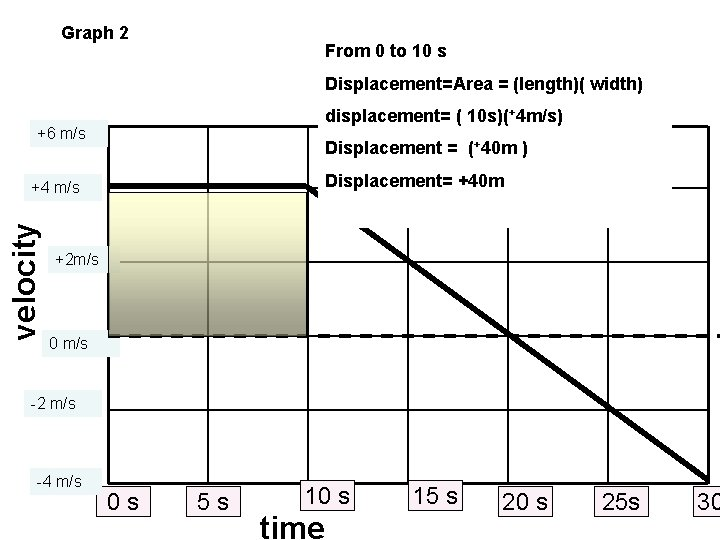Graph 2 From 0 to 10 s Displacement=Area = (length)( width) displacement= ( 10 s)(+4 m/s) +6 m/s Displacement = (+40 m ) Displacement= +40 m velocity +4 m/s +2 m/s 0 m/s -2 m/s -4 m/s 0 s 5 s 10 s time 15 s 20 s 25 s 30Graph 2 From 10 to 20 s Displacement=Area = ½ (base)(height) displacement= ½( 10 s)(+4 m/s) +6 m/s Displacement = ½(+40 m ) Displacement= +20 m velocity +4 m/s +2 m/s 0 m/s -2 m/s -4 m/s 0 s 5 s 10 s time 25 s 20 s 25 s 30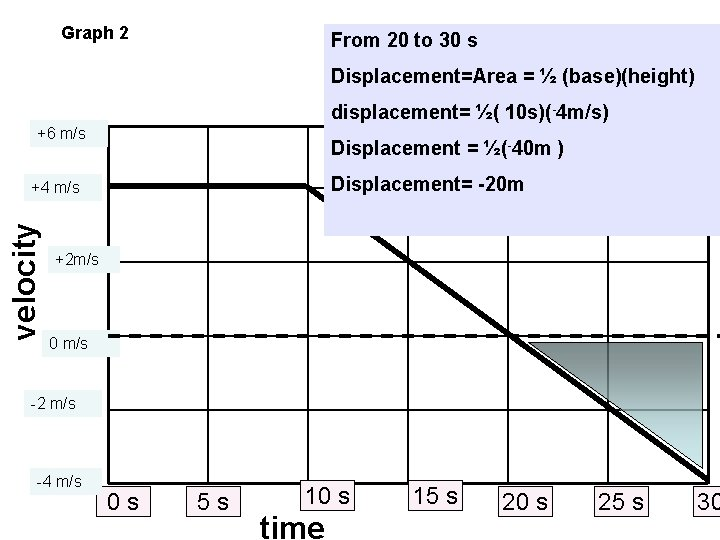Graph 2 From 20 to 30 s Displacement=Area = ½ (base)(height) displacement= ½( 10 s)(-4 m/s) +6 m/s Displacement = ½(-40 m ) Displacement= -20 m velocity +4 m/s +2 m/s 0 m/s -2 m/s -4 m/s 0 s 5 s 10 s time 15 s 20 s 25 s 30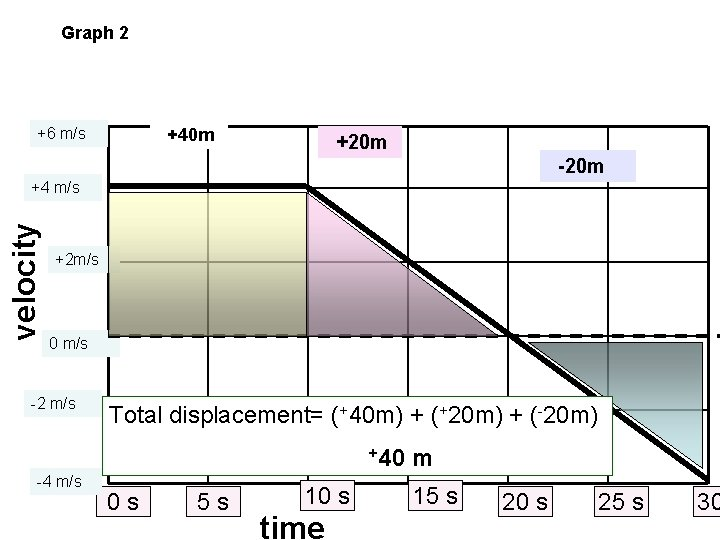Graph 2 +6 m/s +40 m +20 m -20 m velocity +4 m/s +2 m/s 0 m/s -2 m/s Total displacement= (+40 m) + (+20 m) + (-20 m) +40 -4 m/s 0 s 5 s 10 s time m 15 s 20 s 25 s 30Graph #3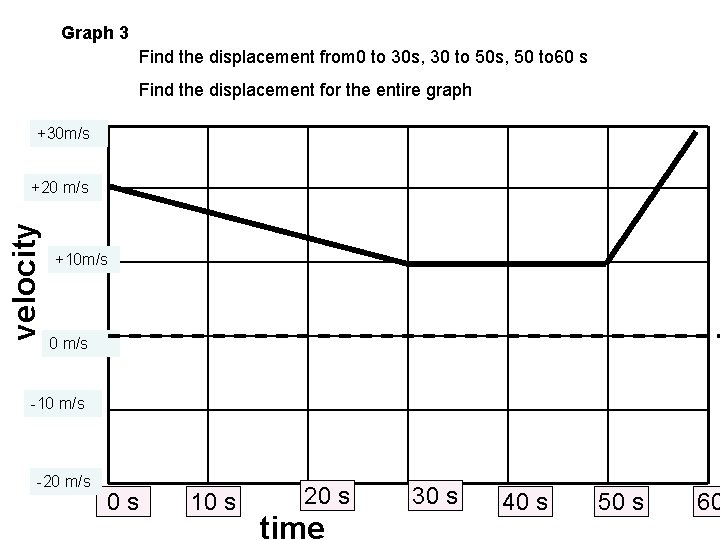Graph 3 Find the displacement from 0 to 30 s, 30 to 50 s, 50 to 60 s Find the displacement for the entire graph +30 m/s velocity +20 m/s +10 m/s 0 m/s -10 m/s -20 m/s 0 s 10 s 20 s time 30 s 40 s 50 s 60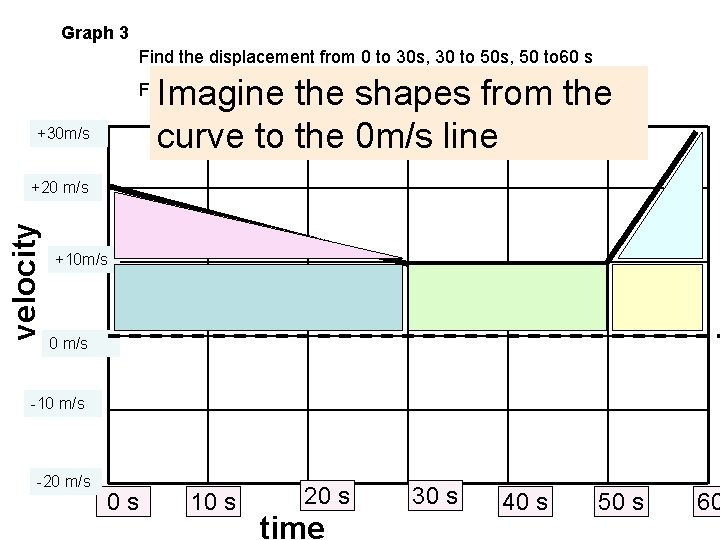Graph 3 Find the displacement from 0 to 30 s, 30 to 50 s, 50 to 60 s Imagine the shapes from the curve to the 0 m/s line Find the displacement for the entire graph +30 m/s velocity +20 m/s +10 m/s 0 m/s -10 m/s -20 m/s 0 s 10 s 20 s time 30 s 40 s 50 s 60Graph 3 From 0 to 30 s Displacement=Area = ½ (base)(height) displacement= ½( 30 s)(+10 m/s) Displacement = ½(+300 m ) Displacement= +150 m +30 m/s velocity +20 m/s From 0 to 30 s Displacement=Area = (length)( width) displacement= ( 30 s)(+10 m/s) Displacement = (+300 m ) Displacement= +300 m +10 m/s 0 m/s -10 m/s Total displacement from 0 to 30 s = (+150 m) + (+300 m) = 450 m -20 m/s 0 s 10 s 20 s time 30 s 40 s 50 s 60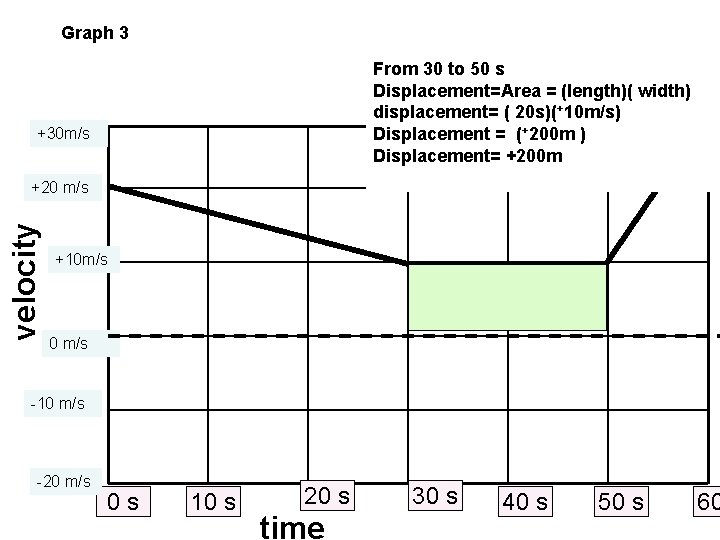Graph 3 From 30 to 50 s Displacement=Area = (length)( width) displacement= ( 20 s)(+10 m/s) Displacement = (+200 m ) Displacement= +200 m +30 m/s velocity +20 m/s +10 m/s 0 m/s -10 m/s -20 m/s 0 s 10 s 20 s time 30 s 40 s 50 s 60Graph 3 From 50 to 60 s Displacement=Area = ½ (base)(height) displacement= ½( 10 s)(+20 m/s) Displacement = ½(+200 m ) Displacement= +100 m +30 m/s velocity +20 m/s From 50 to 60 s Displacement=Area = (length)( width) displacement= ( 10 s)(+10 m/s) Displacement = (+100 m ) Displacement= +100 m +10 m/s 0 m/s Total displacement= (+100 m) + (+100 m) -10 m/s = +200 m -20 m/s 0 s 10 s 20 s time 30 s 40 s 50 s 60Graph 3 Find the displacement from 0 to 30 s, 30 to 50 s, 50 to 60 s Find the displacement for the entire graph +30 m/s +450 m velocity +20 m/s +200 m +10 m/s 0 m/s Total displacement = (+450 m) + (+200 m)= -10 m/s -20 m/s 850 m 0 s 10 s 20 s time 30 s 40 s 50 s 60Graph #4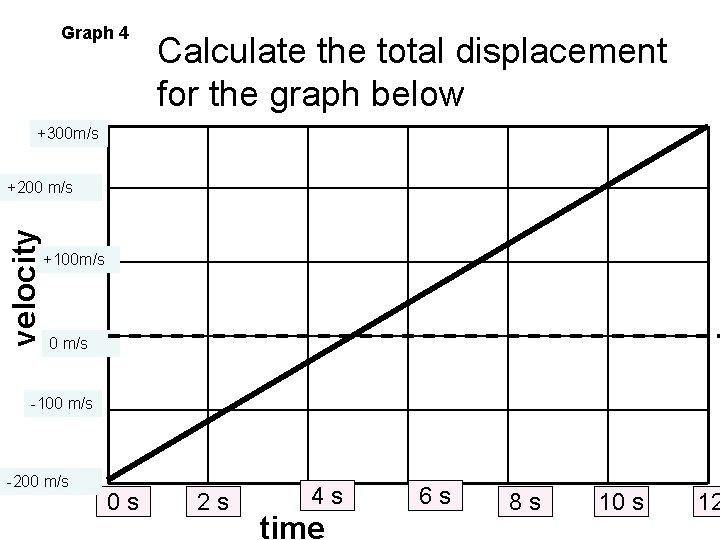Graph 4 Calculate the total displacement for the graph below +300 m/s velocity +200 m/s +100 m/s 0 m/s -100 m/s -200 m/s 0 s 2 s 4 s time 6 s 8 s 10 s 12Graph 4 Calculate the total displacement for the graph below Imagine the shapes from the curve to the 0 m/s line +300 m/s velocity +200 m/s +100 m/s 0 m/s -100 m/s -200 m/s 0 s 2 s 4 s time 6 s 8 s 10 s 12Graph 4 Calculate the total displacement for the graph below +300 m/s velocity +200 m/s From 0 to 5 s Displacement=Area = ½ (base) (height) displacement= ½( 5 s)(-200 m/s) Displacement = ½(-1000 m ) Displacement= -500 m +100 m/s 0 m/s -100 m/s -200 m/s 0 s 2 s 4 s time 6 s 8 s 10 s 12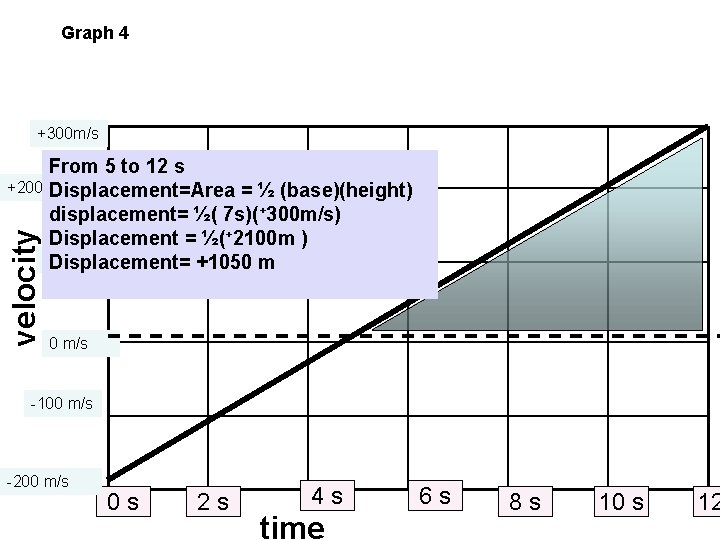Graph 4 +300 m/s velocity From 5 to 12 s +200 m/s Displacement=Area = ½ (base)(height) displacement= ½( 7 s)(+300 m/s) Displacement = ½(+2100 m ) +100 m/s Displacement= +1050 m 0 m/s -100 m/s -200 m/s 0 s 2 s 4 s time 6 s 8 s 10 s 12Graph 4 Calculate the total displacement for the graph below +300 m/s +1050 m velocity +200 m/s -500 m +100 m/s 0 m/s Total displacement = (-500 m) + (+1050 m) = +550 m -100 m/s -200 m/s 0 s 2 s 4 s time 6 s 8 s 10 s 12…But what if the curve on a graph is actually a curve?This powerpoint was kindly donated to www. worldofteaching. com http: //www. worldofteaching. com Is home to well over a thousand powerpoints submitted by teachers. This a free site. Please visit and I hope it will help in your teaching